Check Full Chapter Explained - Continuity and Differentiability - Application of Derivatives (AOD) Class 12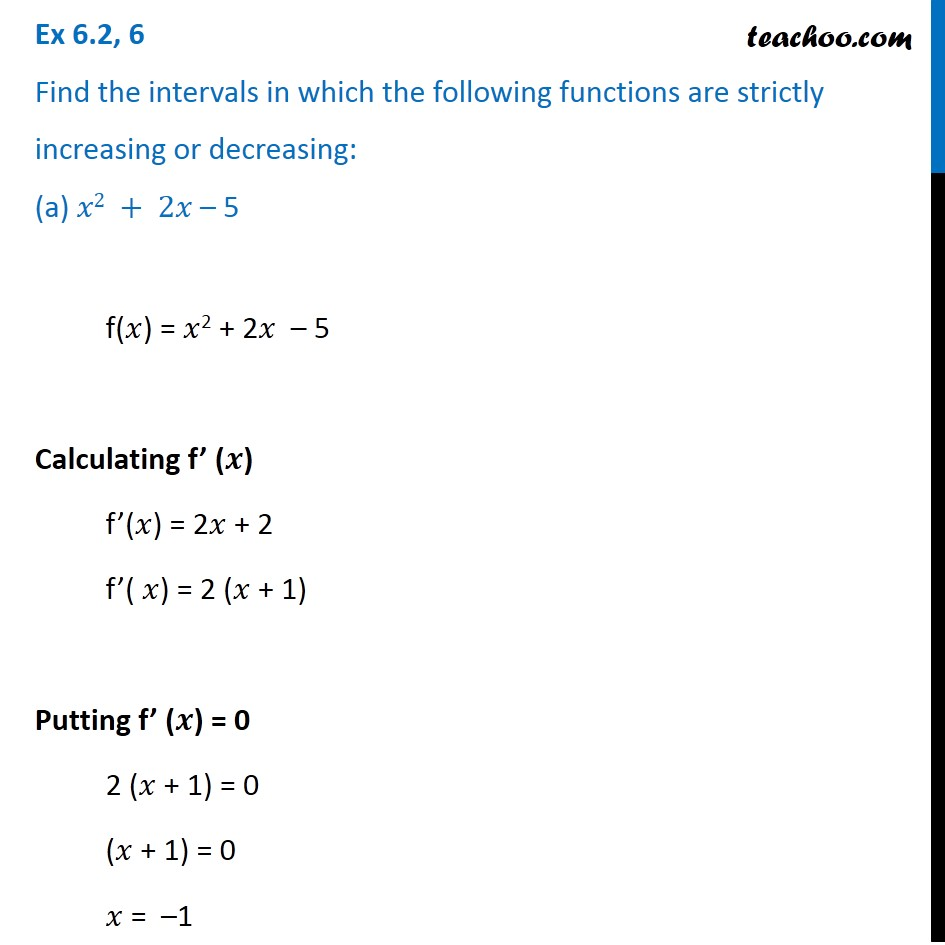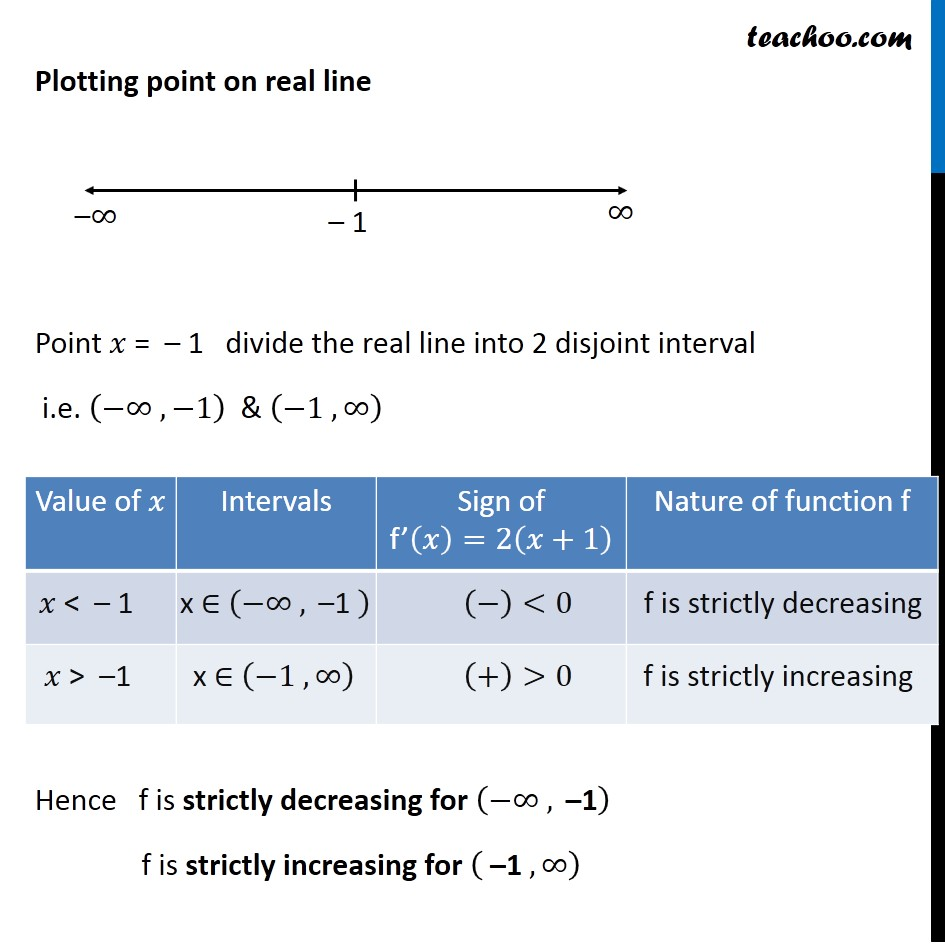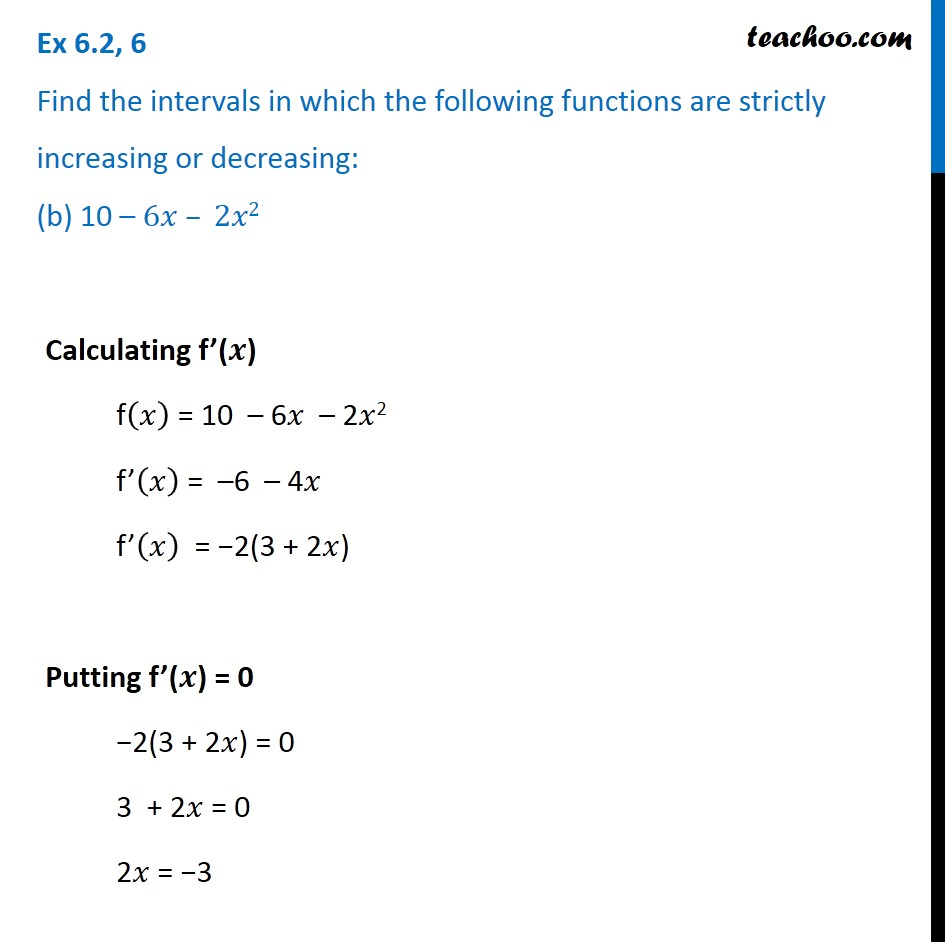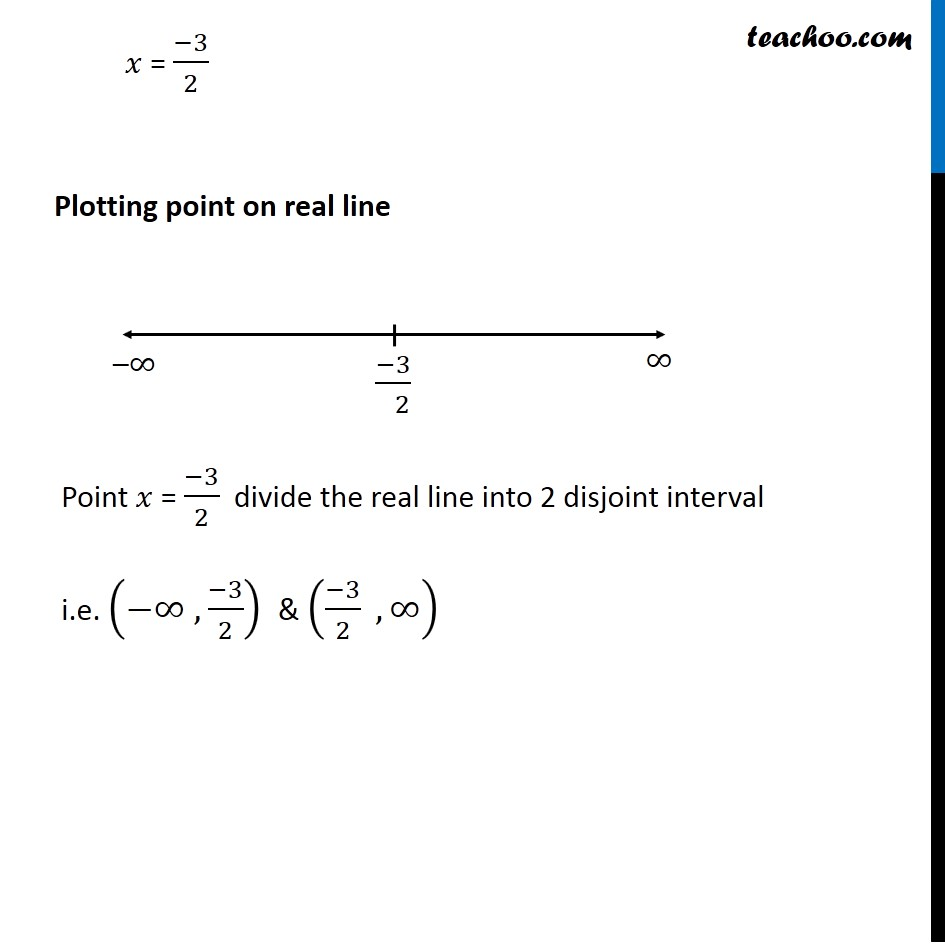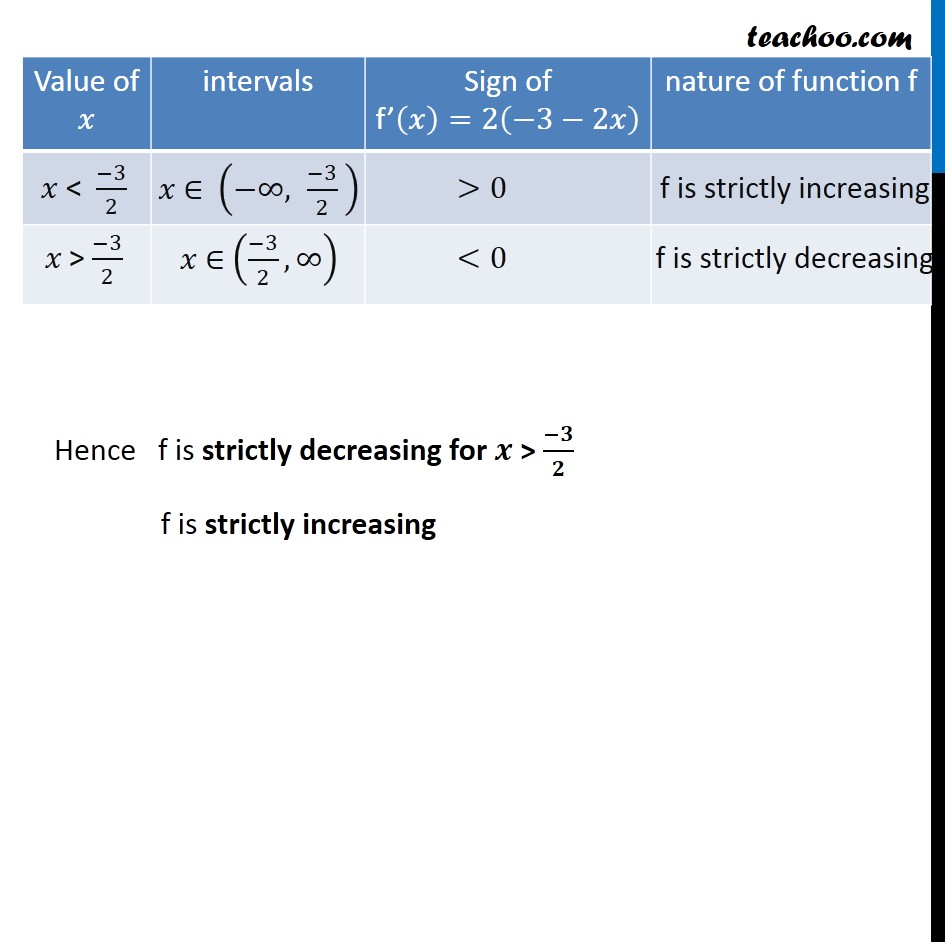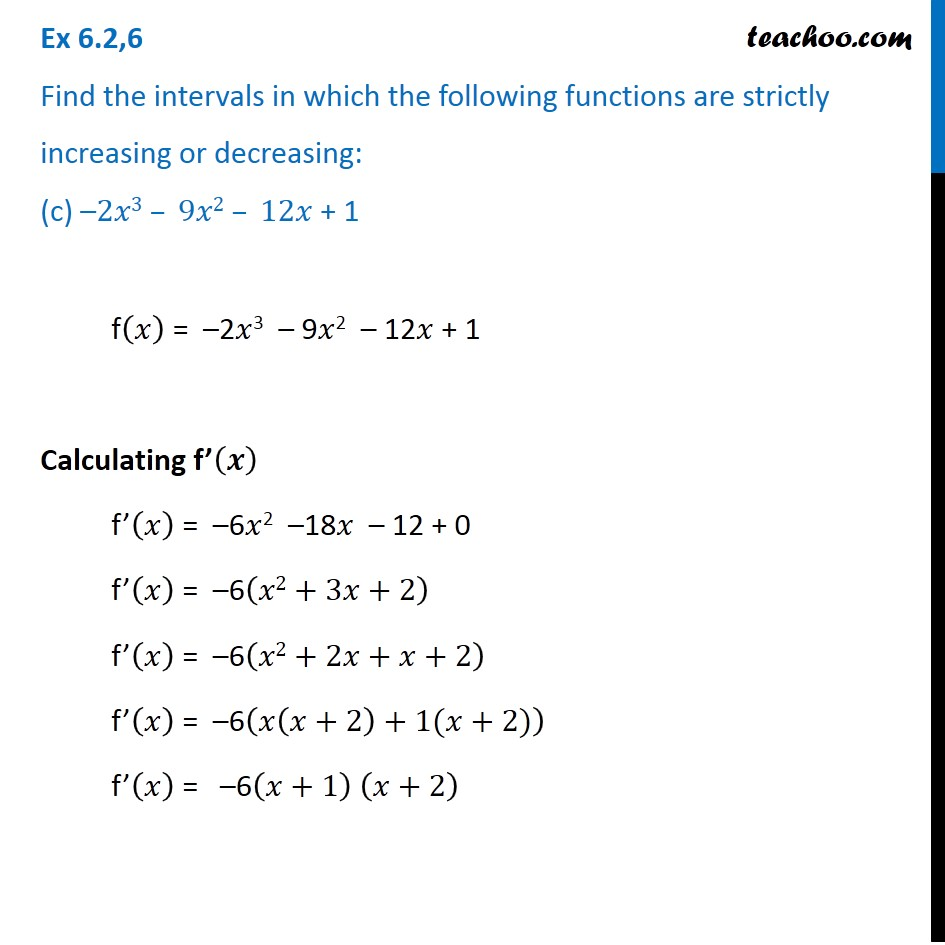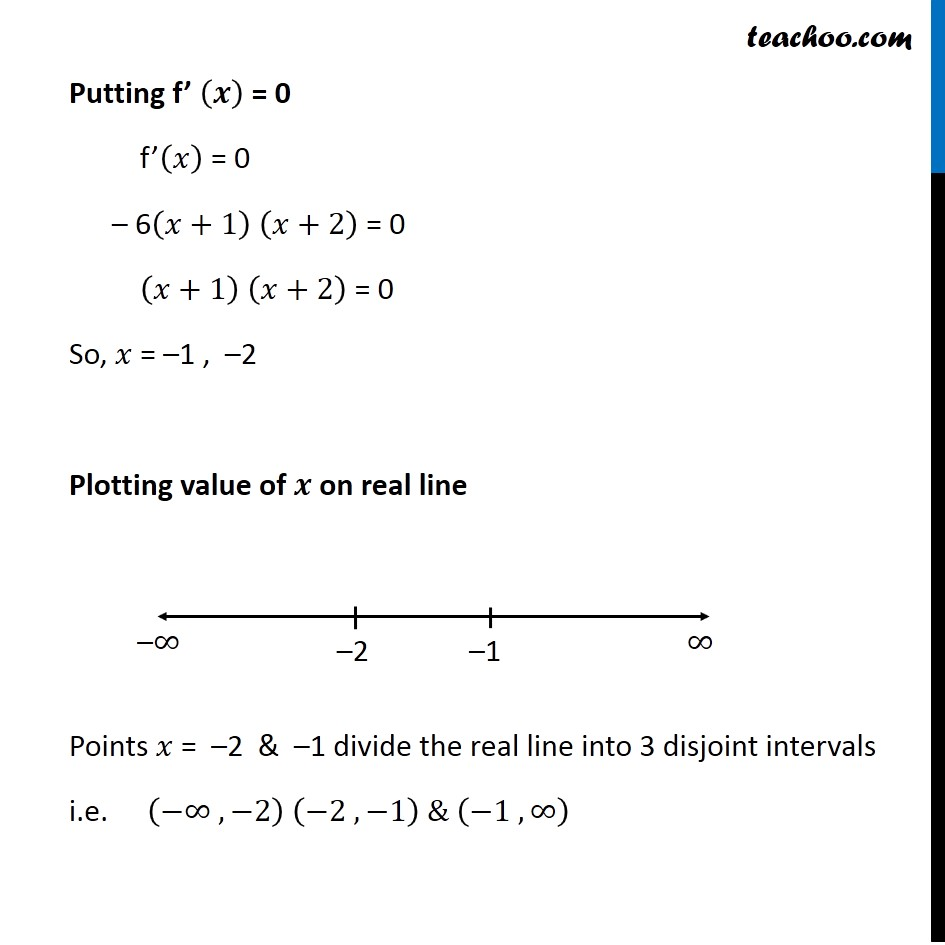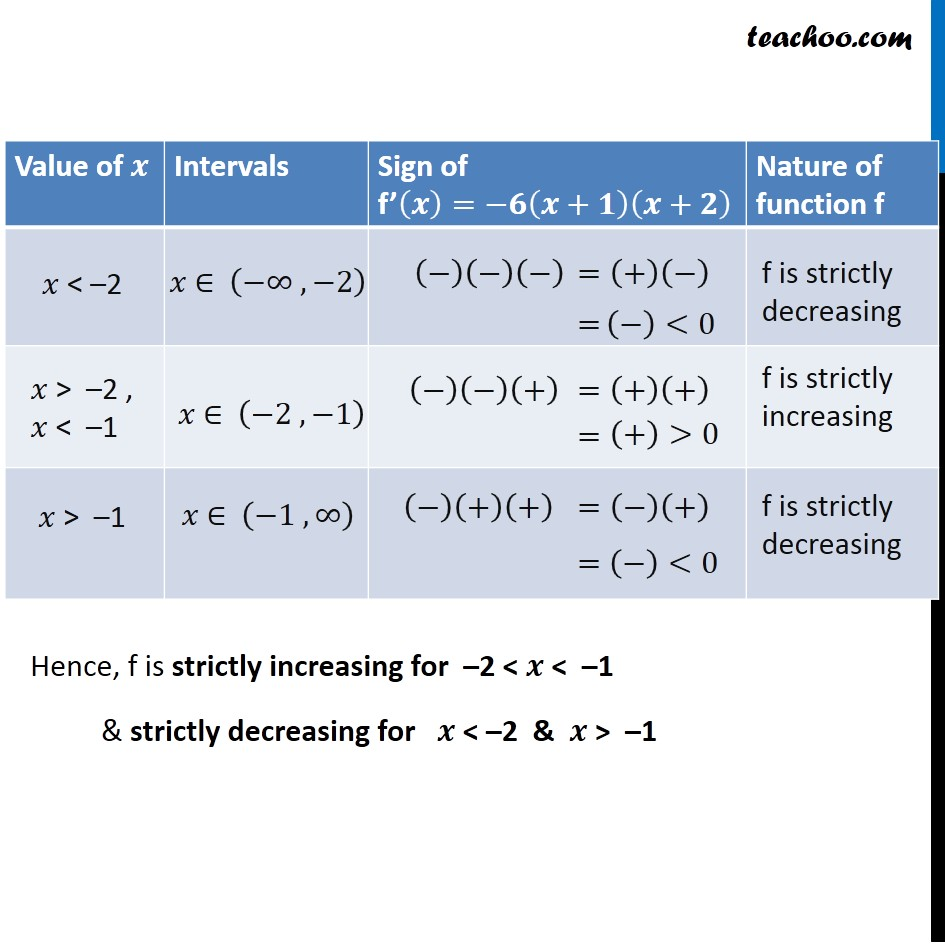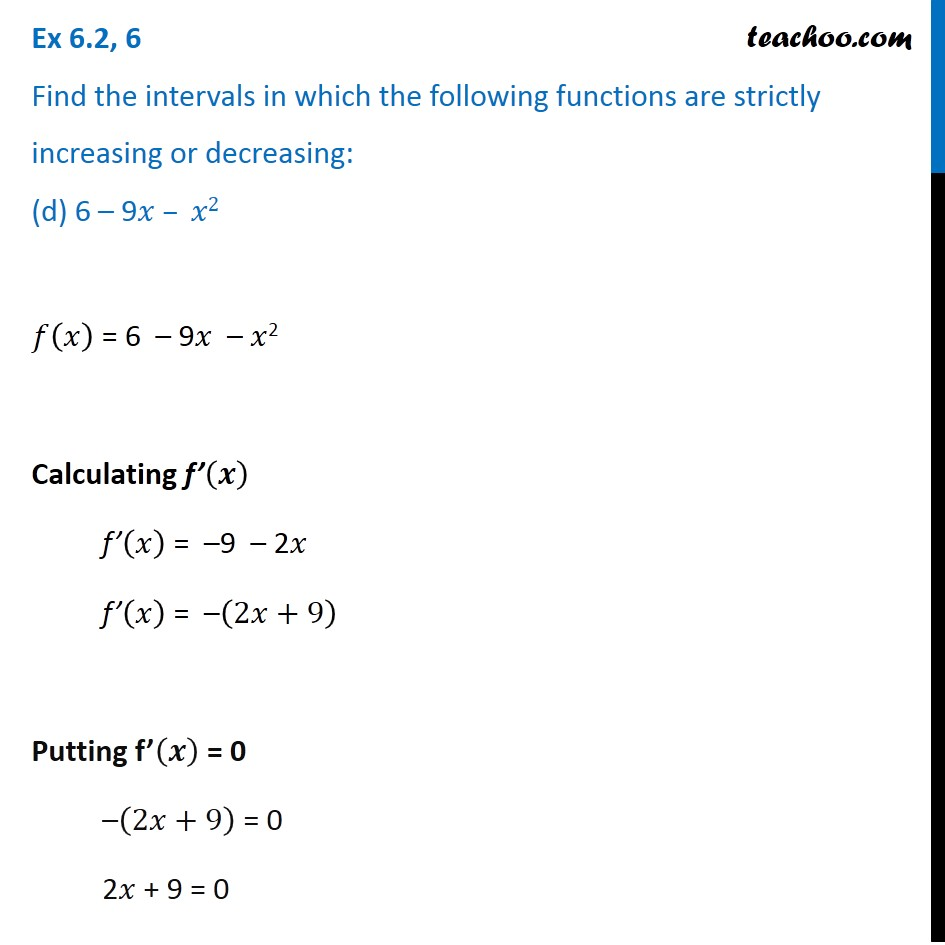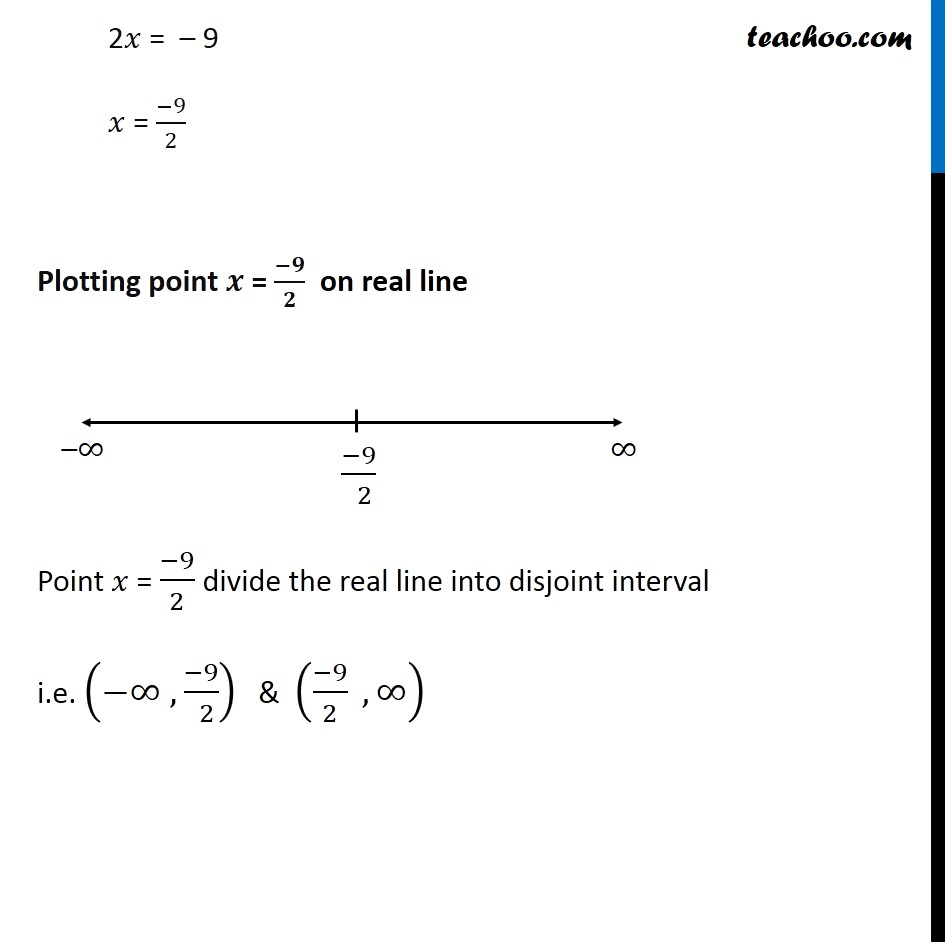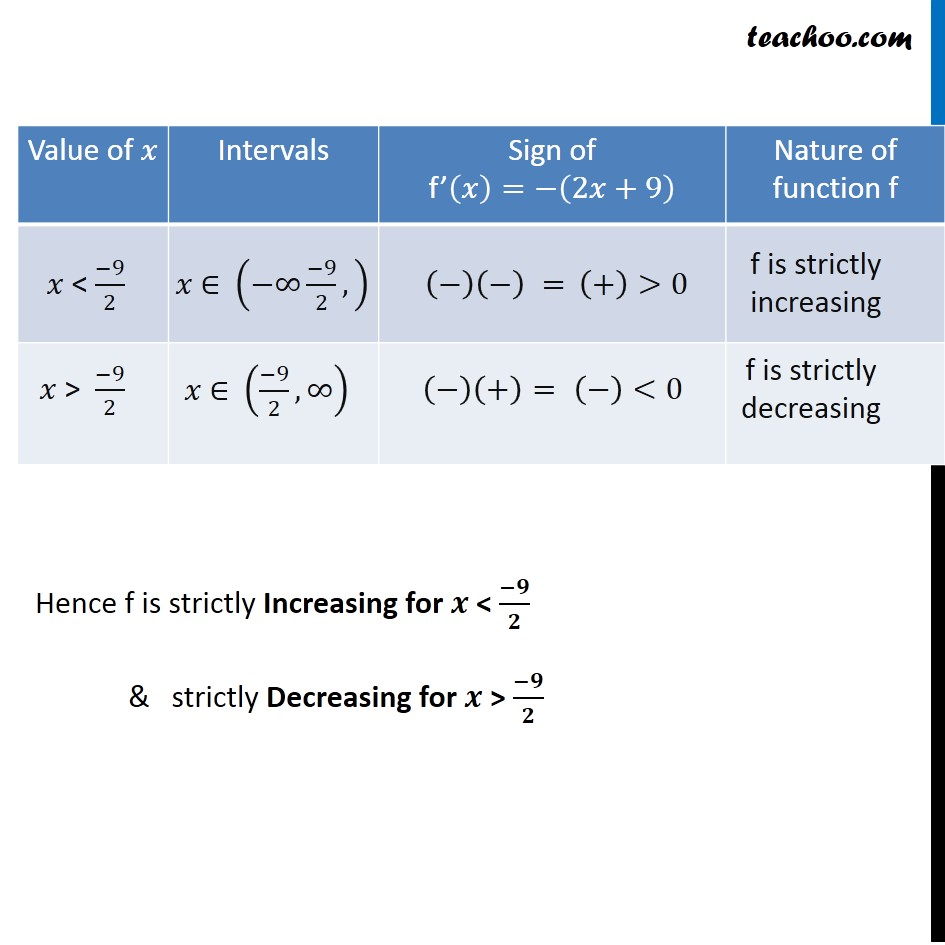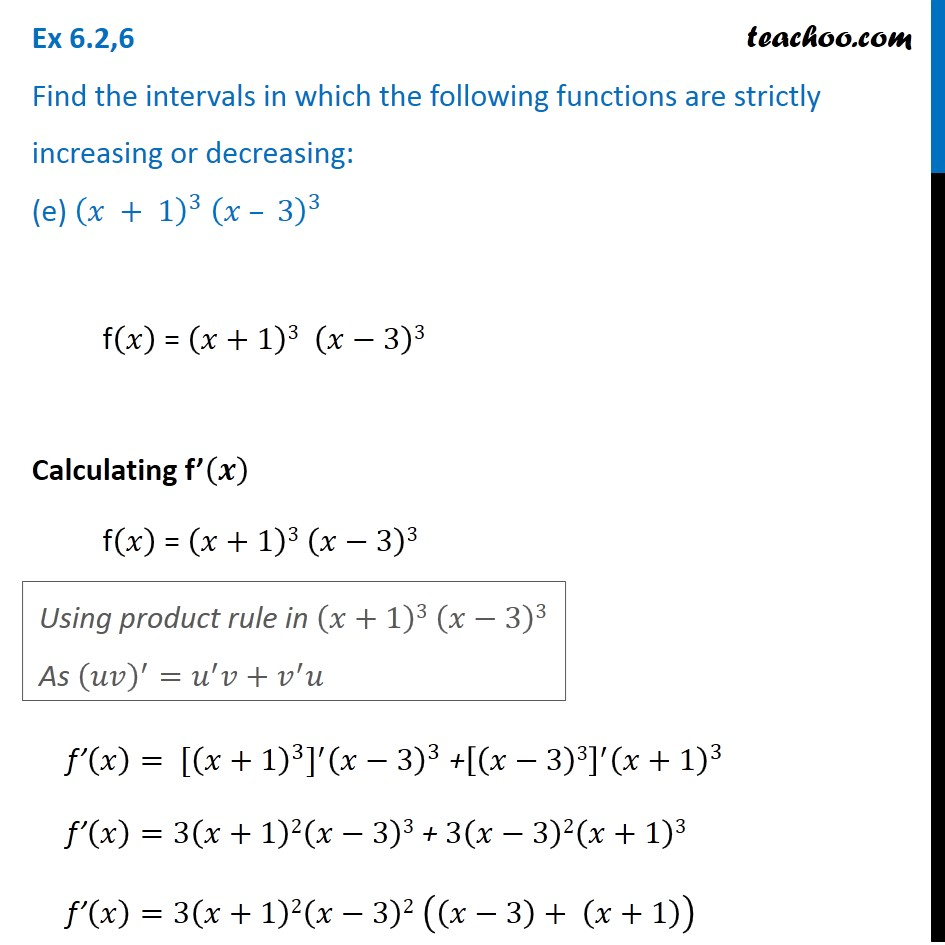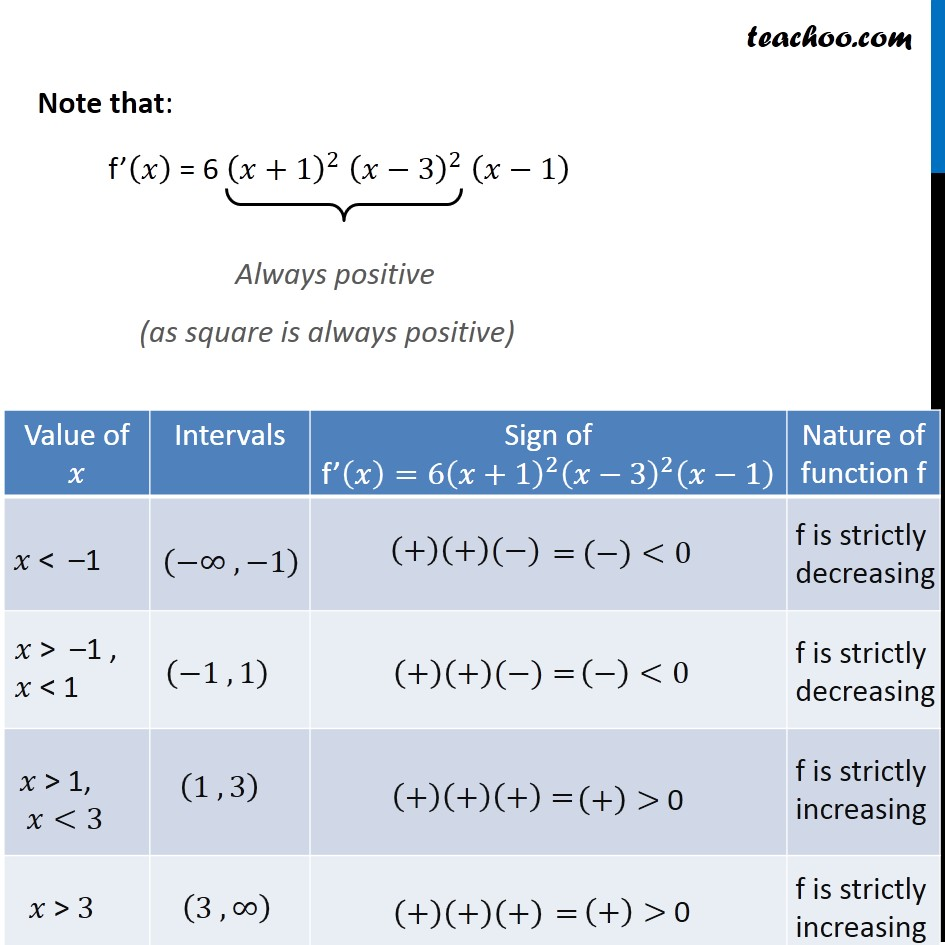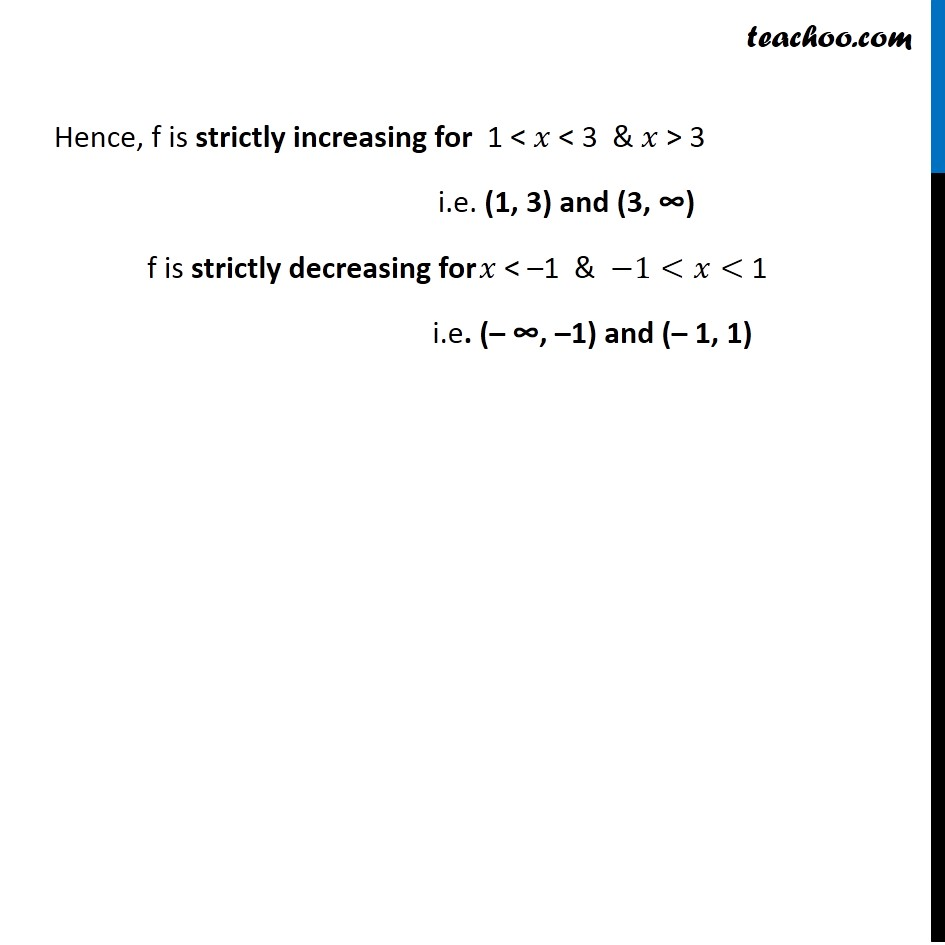1. Chapter 6 Class 12 Application of Derivatives
2. Serial order wise
3. Ex 6.2

Transcript

Ex 6.2, 6 Find the intervals in which the following functions are strictly increasing or decreasing: (a) 𝑥2 + 2𝑥 – 5 f(𝑥) = 𝑥2 + 2𝑥 – 5 Calculating f’ (𝒙) f’(𝑥) = 2𝑥 + 2 f’( 𝑥) = 2 (𝑥 + 1) Putting f’ (𝒙) = 0 2 (𝑥 + 1) = 0 (𝑥 + 1) = 0 𝑥 = –1 Plotting point on real line Point 𝑥 = – 1 divide the real line into 2 disjoint interval i.e. (−∞ , −1) & (−1 , ∞) Hence f is strictly decreasing for (−∞ ," –1" ) f is strictly increasing for ( "–1" ,∞) Ex 6.2, 6 Find the intervals in which the following functions are strictly increasing or decreasing: (b) 10 – 6𝑥 – 2𝑥2 Calculating f’(𝒙) f(𝑥) = 10 – 6𝑥 – 2𝑥2 f’(𝑥) = –6 – 4𝑥 f’(𝑥) = −2(3 + 2𝑥) Putting f’(𝒙) = 0 −2(3 + 2𝑥) = 0 3 + 2𝑥 = 0 2𝑥 = −3 𝑥 = (−3)/2 Plotting point on real line Point 𝑥 = (−3)/2 divide the real line into 2 disjoint interval i.e. (−∞ , (−3)/2) & ((−3)/2 , ∞) Hence f is strictly decreasing for 𝒙 > (−𝟑)/𝟐 f is strictly increasing Ex 6.2,6 Find the intervals in which the following functions are strictly increasing or decreasing: (c) –2𝑥3 – 9𝑥2 – 12𝑥 + 1 f(𝑥) = –2𝑥3 – 9𝑥2 – 12𝑥 + 1 Calculating f’(𝒙) f’(𝑥) = –6𝑥2 –18𝑥 – 12 + 0 f’(𝑥) = –6(𝑥2+3𝑥+2) f’(𝑥) = –6(𝑥2+2𝑥+𝑥+2) f’(𝑥) = –6(𝑥(𝑥+2)+1(𝑥+2)) f’(𝑥) = –6(𝑥+1) (𝑥+2) Putting f’ (𝒙) = 0 f’(𝑥) = 0 – 6(𝑥+1) (𝑥+2) = 0 (𝑥+1) (𝑥+2) = 0 So, 𝑥 = –1 , –2 Plotting value of 𝒙 on real line Points 𝑥 = –2 & –1 divide the real line into 3 disjoint intervals i.e. (−∞ , −2) (−2 , −1) & (−1 , ∞) Hence, f is strictly increasing for –2 < 𝒙 < –1 & strictly decreasing for 𝒙 < –2 & 𝒙 > –1 Ex 6.2, 6 Find the intervals in which the following functions are strictly increasing or decreasing: (d) 6 – 9𝑥 – 𝑥2 𝑓(𝑥) = 6 – 9𝑥 – 𝑥2 Calculating f’(𝒙) f’(𝑥) = –9 – 2𝑥 f’(𝑥) = –(2𝑥+9) Putting f’(𝒙) = 0 –(2𝑥+9) = 0 2𝑥 + 9 = 0 2𝑥 = – 9 𝑥 = (−9)/2 Plotting point 𝒙 = (−𝟗)/𝟐 on real line Point 𝑥 = (−9)/2 divide the real line into disjoint interval i.e. (−∞ , (−9)/( 2)) & ((−9)/2 , ∞) Hence f is strictly Increasing for 𝒙 < (−𝟗)/𝟐 & strictly Decreasing for 𝒙 > (−𝟗)/𝟐 Ex 6.2,6 Find the intervals in which the following functions are strictly increasing or decreasing: (e) (𝑥 + 1)^3 (𝑥 – 3)^3 f(𝑥) = (𝑥+1)3 (𝑥−3)3 Calculating f’(𝒙) f(𝑥) = (𝑥+1)3 (𝑥−3)3 f’(𝑥)= 〖[(𝑥+1)^3]〗^′ (𝑥−3)^3 +[(𝑥−3)3]^′ (𝑥+1)^3 f’(𝑥)=3(𝑥+1)2(𝑥−3)3 + 3(𝑥−3)2(𝑥+1)3 f’(𝑥)=3(𝑥+1)2(𝑥−3)2 ((𝑥−3)+ (𝑥+1)) Using product rule in (𝑥+1)3 (𝑥−3)3 As (𝑢𝑣)^′=𝑢^′ 𝑣+𝑣^′ 𝑢 f’(𝑥)=3(𝑥+1)2(𝑥−3)2 (2𝑥−2) f’(𝑥)= 6(𝑥+1)2 (𝑥−3)2 (𝑥−1) Putting f’(𝒙)=𝟎 6(𝑥+1)2 (𝑥−3)2 (𝑥−1) = 0 Hence, 𝑥 = –1 , 1 , & 3 Plotting values of x on real line. The point x = –1 , 1 & 3 divide the line segment into 4 disjoint intervals i.e. (−∞ , −1) (−1 , 1) (1 , 3) & (3 , ∞) Note that: f’(𝑥) = 6 (𝑥+1)^2 (𝑥−3)^2 (𝑥−1) Always positive (as square is always positive) Hence, f is strictly increasing for 1 < 𝑥 < 3 & 𝑥 > 3 i.e. (1, 3) and (3, ∞) f is strictly decreasing for 𝑥 < –1 & −1<𝑥< 1 i.e. (– ∞, –1) and (– 1, 1)

Ex 6.2